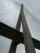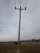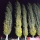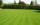# Seamstress

The seamstress cut the fabric into 3 parts. The first part was the eighth fabric, the second part was three-fifths of the fabric and the third part had a length of 66 cm. Calculate the original length of the fabric.

Result

x =  240 cm

#### Solution:

x/8 + 3/5 x + 66 = x

11x = 2640

x = 240

Calculated by our simple equation calculator.

Leave us a comment of example and its solution (i.e. if it is still somewhat unclear...):

Showing 0 comments:Be the first to comment!#### To solve this example are needed these knowledge from mathematics:

Need help calculate sum, simplify or multiply fractions? Try our fraction calculator. Do you have a linear equation or system of equations and looking for its solution? Or do you have quadratic equation? Do you want to convert length units?

## Next similar examples:

1. Bridge piersOne quarter of the bridge pier is sunk into the ground. Two thirds are in the water. Protruding above the water is 1.20 m long. Determine the height of bridge piers.
2. Cleaning windowsCleaning company has to wash all the windows of the school. The first day washes one-sixth of the windows of the school, the next day three more windows than the first day and the remaining 18 windows washes on the third day. Calculate how many windows ha
3. Iron poleThe iron pole is in the ground 2/5 of its length, partly above the ground 1/3 is yellow, and the unpainted section is 6 m long. How long is the entire column?
4. FractionsThree-quarters of an unknown number are 4/5. What is 5/6 of this unknown number?
5. Class 8.AThree quarters of class 8.A went skiing. Of those who remained at home one third was ill and the remaining six were on math olympic. How many students have class 8.A?
6. PearsThere were pears in the basket, I took two-fifths of them, and left six in the basket. How many pears did I take?
7. Simple equationSolve for x: 3(x + 2) = x - 18
8. PoplarHow tall is a poplar by the river, if we know that 1/5 of its total height is a trunk, 1/10th of the height is the root and 35m from the trunk to the top of the poplar?
9. Simple equation 6Solve equation with one variable: X/2+X/3+X/4=X+4
10. EquationSolve the equation: 1/2-2/8 = 1/10; Write the result as a decimal number.
11. UN 1If we add to an unknown number his quarter, we get 210. Identify unknown number.
12. Equation with fractionsSolve equation: ? It is equation with fractions.
13. Equation with xSolve the following equation: 2x- (8x + 1) - (x + 2) / 5 = 9
14. Unknown numberIdentify unknown number which 1/5 is 40 greater than one tenth of that number.
15. Unknown numberI think the number - its sixth is 3 smaller than its third.
16. EqnSolve equation with fractions: 2x/3-50=40+x/4
17. Mr. HappyMr. Happy planted 36.6 meters square gardens grass; It's a third of the garden more than half of the garden. What is square area of this garden?